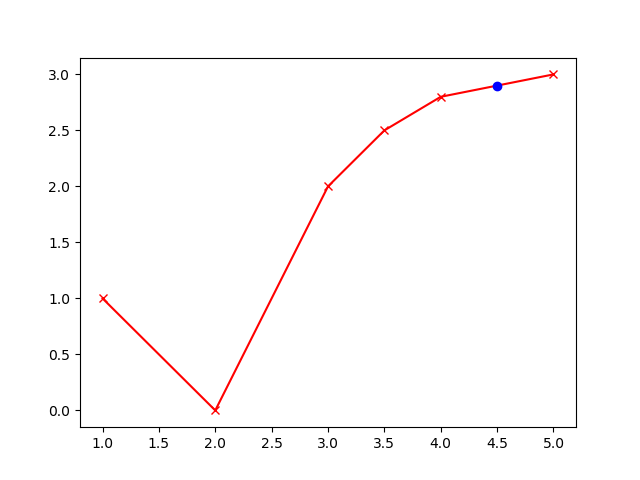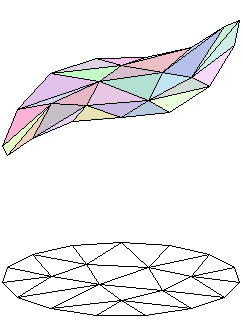## Apps.PiecewiseLinear History

February 14, 2019, at 03:37 PM by 10.37.111.106 -

#### Piece-wise Function with Lookup Object

February 14, 2019, at 03:36 PM by 10.37.111.106 -
Changed line 19 from:

(:html:)<font size=2><pre>

to:

(:source lang=python:)

Changed lines 47-49 from:

</pre></font> (:htmlend:)

to:

(:sourceend:)

Changed line 53 from:

(:html:)<font size=2><pre>

to:

(:source lang=python:)

Changed lines 107-109 from:

</pre></font> (:htmlend:)

to:

(:sourceend:)

Changed line 167 from:

(:html:)<font size=2><pre>

to:

(:source lang=python:)

Changed lines 207-208 from:

</pre></font> (:htmlend:)

to:

(:sourceend:)

Changed lines 115-116 from:to:Deleted line 117:

from scipy import optimize

#### PWL in GEKKO Python(:source lang=python:) from scipy import optimize import matplotlib.pyplot as plt from gekko import GEKKO import numpy as np

m = GEKKO() m.options.SOLVER = 1

xz = m.FV(value = 4.5) yz = m.Var()

xp_val = np.array([1, 2, 3, 3.5, 4, 5]) yp_val = np.array([1, 0, 2, 2.5, 2.8, 3]) xp = [m.Param(value=xp_val[i]) for i in range(6)] yp = [m.Param(value=yp_val[i]) for i in range(6)]

x = [m.Var(lb=xp[i],ub=xp[i+1]) for i in range(5)] x.lower = -1e20 x[-1].upper = 1e20

1. Variables

slk_u = [m.Var(value=1,lb=0) for i in range(4)] slk_l = [m.Var(value=1,lb=0) for i in range(4)]

1. Intermediates

slope = [] for i in range(5):

    slope.append(m.Intermediate((yp[i+1]-yp[i]) / (xp[i+1]-xp[i])))


y = [] for i in range(5):

    y.append(m.Intermediate((x[i]-xp[i])*slope[i]))


for i in range(4):

    m.Obj(slk_u[i] + slk_l[i])


m.Equation(xz == x + slk_u) for i in range(3):

    m.Equation(xz == x[i+1] + slk_u[i+1] - slk_l[i])


m.Equation(xz == x - slk_l)

m.Equation(yz == yp + y + y + y + y + y)

m.solve()

plt.plot(xp,yp,'rx-',label='PWL function') plt.plot(xz,yz,'bo',label='Data') plt.show() (:sourceend:)

July 06, 2018, at 09:49 PM by 45.56.3.173 -

#### APMonitor PWL Object

Changed lines 20-37 from:

Model pwl

to:
  File p.txt
1,   1
2,   0
3,   2
3.5, 2.5
4,   2.8
5,   3
End File

Objects
p = pwl
End Objects

Connections
x = p.x
y = p.y
End Connections

Deleted lines 40-58:
    ! data points
xp = 1
yp = 1

xp = 2
yp = 0

xp = 3
yp = 2

xp = 3.5
yp = 2.5

xp = 4
yp = 2.8

xp = 5
yp = 3

Deleted lines 43-47:
    ! piece-wise linear segments
x             <=xp
x[2:4] >xp[2:4], <=xp[3:5]
x   >xp

Deleted lines 45-48:
    ! slack variables
slk_u[1:4]
slk_l[2:5]

Changed lines 47-92 from:
to:

</pre></font> (:htmlend:)

#### Equivalent Problem with Slack Variables

(:html:)<font size=2><pre>

  Parameters
! independent variable
x = 6.0

! data points
xp = 1
yp = 1

xp = 2
yp = 0

xp = 3
yp = 2

xp = 3.5
yp = 2.5

xp = 4
yp = 2.8

xp = 5
yp = 3
End Parameters

Variables
! piece-wise linear segments
x             <=xp
x[2:4] >xp[2:4], <=xp[3:5]
x   >xp

! dependent variable
y

! slack variables
slk_u[1:4]
slk_l[2:5]
End Variables

Deleted line 107:

End Model

July 31, 2017, at 07:56 PM by 152.7.224.8 -
Changed line 11 from:

In Situ Adaptive Tabulation (ISAT) is an example of a multi-dimensional piecewise linear approximation. The piecewise linear segments are built dynamically as new data becomes available. This way, only regions that are accessed in practice contribute to the function approximation.

to:

In Situ Adaptive Tabulation (ISAT) is an example of a multi-dimensional piecewise linear approximation. The piecewise linear segments are built dynamically as new data becomes available. This way, only regions that are accessed in practice contribute to the function approximation.

February 13, 2013, at 05:09 PM by 69.169.188.188 -
    minimize slk_u[1:4]
minimize slk_l[2:5]

Changed line 83 from:

File *.m.csv

to:

File m.csv

August 28, 2010, at 04:16 AM by 206.180.155.75 -
  End Equations


End Model </pre></font> (:htmlend:)

(:html:)<font size=2><pre>

# create the csv file

File *.m.csv

  input,  y,  y
1,     2,    4
3,     4,    6
5,    -5,   -7
-1,     1,  0.5


End File

# define lookup object m

Objects

  m = lookup


End Objects

# connect m properties with model parameters

Connections

  x = m.input
y = m.y
y = m.y


End Connections

# simple model

Model n

  Parameters
x = 1
y
y
End Parameters

Variables
y
End Variables

Equations
y = y+y

March 06, 2010, at 09:45 AM by 206.180.155.75 -
Changed line 17 from:

(:html:)<font size=1><pre>

to:

(:html:)<font size=2><pre>

Changed line 71 from:

(:htmlend:)

to:

(:htmlend:)

April 23, 2009, at 04:46 PM by 158.35.225.231 -
Changed lines 15-71 from:
to:

(:html:)<font size=1><pre> Model pwl

  Parameters
! independent variable
x = 6.0

! data points
xp = 1
yp = 1

xp = 2
yp = 0

xp = 3
yp = 2

xp = 3.5
yp = 2.5

xp = 4
yp = 2.8

xp = 5
yp = 3
End Parameters

Variables
! piece-wise linear segments
x             <=xp
x[2:4] >xp[2:4], <=xp[3:5]
x   >xp

! dependent variable
y

! slack variables
slk_u[1:4]
slk_l[2:5]
End Variables

Intermediates
slope[1:5] = (yp[2:6]-yp[1:5]) / (xp[2:6]-xp[1:5])
y[1:5] = (x[1:5]-xp[1:5])*slope[1:5]
End Intermediates

Equations
x = x   + slk_u
x = x[2:4] + slk_u[2:4] - slk_l[2:4]
x = x                - slk_l

y = yp + y + y + y + y + y
End Equations


End Model </pre></font> (:htmlend:)

February 23, 2009, at 02:45 PM by 158.35.225.228 -

## Piece-wise Linear ApproximationA piece-wise linear function is an approximation of a nonlinear relationship. For more nonlinear relationships, additional linear segments are added to refine the approximation.

As an example, the piecewise linear form is often used to approximate valve characterization (valve position (% open) to flow). This is a single input-single output function approximation.

In Situ Adaptive Tabulation (ISAT) is an example of a multi-dimensional piecewise linear approximation. The piecewise linear segments are built dynamically as new data becomes available. This way, only regions that are accessed in practice contribute to the function approximation.